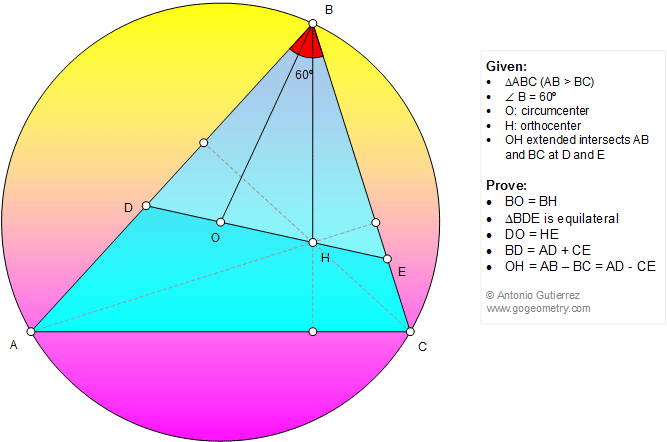# Geometry Problem 1161: Scalene Triangle, 60 Degrees, Angle, Orthocenter, Circumcenter, Equilateral Triangle, Congruence. Level: School, College, Mathematics Education

< PREVIOUS PROBLEM  |  NEXT PROBLEM >

 The figure below shows a triangle ABC (AB > BC) with orthocenter H and circumcenter O such that angle ABC = 60 degrees. Line OH extended intersects AB and BC at D and E, respectively. Prove that (1) BO = BH, (2) triangle BDE is equilateral, (3) DO = HE, (4) BD = AD + CE, (5) OH = AB - BC = AD - CE.See Geometry art of problem 1161

 Home | Search | Geometry | Problems | All Problems | Open Problems | Visual Index | 10 Problems | Problems Art Gallery | Art | 1161-1170 | Triangles | Circle | Equilateral triangle | Circumcenter | Orthocenter | Congruence | 60 degrees | Last updated: Nov 1 2015